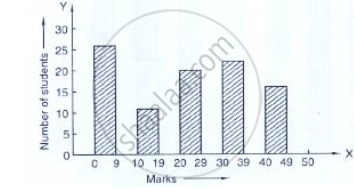# The Bar Graph Indicating the Marks Obtained Out of 50 in Mathematics Paper by 100 Students. Read the Bar Graph (I) It is Decided to Distribute Work Books on Mathematics to the Students Obtaining - Mathematics

Given below (Fig. below) is the bar graph indicating the marks obtained out of 50 in mathematics paper by 100 students. Read the bar graph and answer the following questions:(i) It is decided to distribute work books on mathematics to the students obtaining less than 20 marks, giving one workbook to each of such students. If a work book
costs Rs 5, what sum is required to buy the work books?
(ii) Every student belonging to the highest mark group is entitled to get a prize of Rs. 10. How much amount of money is required for distributing the prize money?
(iii) Every student belonging to the lowest mark—group has to solve 5 problems per day. How many problems, in all, will be solved by the students of this group per day?
(iv) State whether true or false.
a. 17% students have obtained marks ranging from 40 to 49.
b. 59 students have obtained marks ranging from 10 to 29.
(v) What is the number of students getting less than 20 marks?
(vi) What is the number of students getting more than 29 marks?
(vii) What is the number of students getting marks between 9 and 40?
(viii) What is the number of students belonging to the highest mark group?
(ix) What is the number of students obtaining more than 19 marks?

#### Solution

(i) Total number of students obtaining less than 20 marks = 27 + 12 = 39.
The cost off one – work book = Rs 5.
∴ The cost of 30 work books 39 × 5
= Rs 195.
(ii) The number of students belonging to the highest mark group = 17
The cost of a prize = 10.
∴ The cost of 17 prizes =10×17
=Rs 170
(iii) The number of students belonging to the lowest mark group =27
∴ The number of problems solved by 1 student = 5
The total number of problems solved by 27 students 5 × 27
=135.
(iv) (a) Total number of students = 100
The number of students in range 40-49=17.

%of students obtaining marks ranging
40 - 49 = 17/100×100
=17%
So, the given statement is true.
(b) The number of students in range 10-29 =12 =120 = 32.
Percentage of students obtaining marks ranging

10 - 29 = 32/100× 100 = 32%
So, the given statement is false (False).
(v) No. of students getting more than 20 marks = 39
(vi) Total no. of students getting more than 29 marks = 41
(vii) The number of students getting marks between 9 and 40 =12+20+24 = 56.
(viii) The number of students belonging to the highest mark group = 17.
(ix) The number of students obtaining more than 19 marks =100-27-12 = 61

Concept: Graphical Representation of Data
Is there an error in this question or solution?

#### APPEARS IN

RD Sharma Mathematics for Class 9
Chapter 23 Graphical Representation of Statistical Data
Exercise 23.1 | Q 7 | Page 10

Share